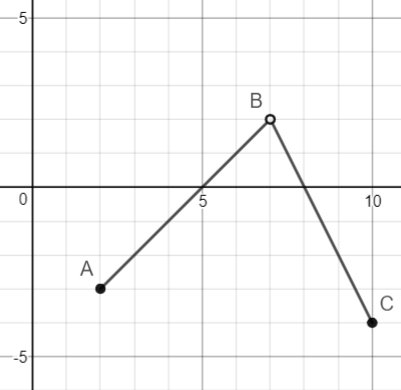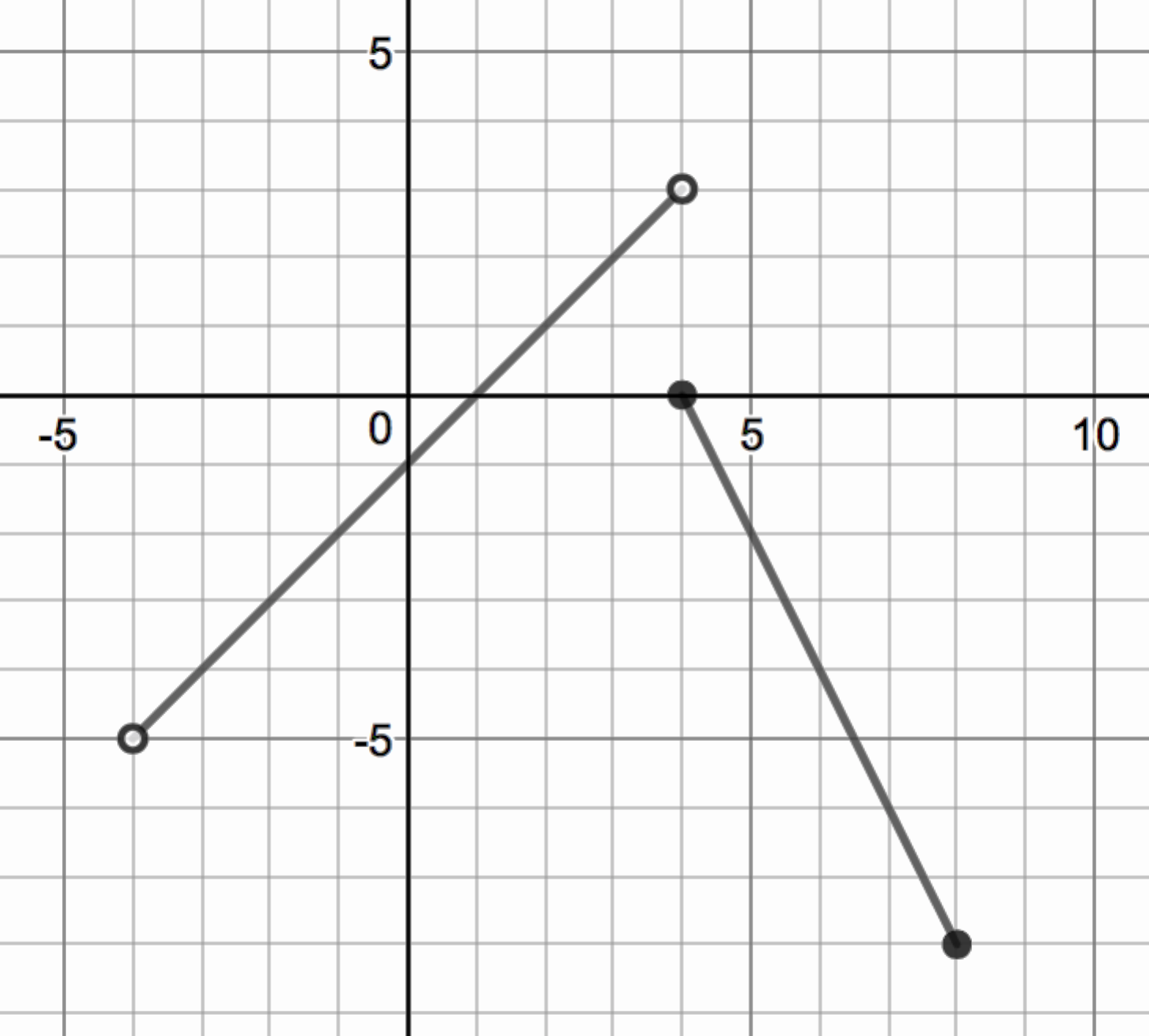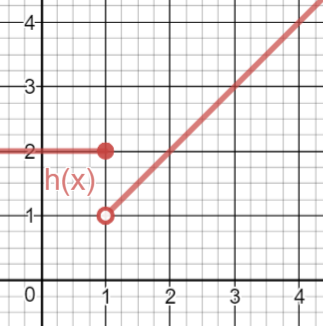# Limits and Continuity

## Objective

Find limits, including left- and right-hand limits, on a function given graphically.

## Criteria for Success

?

1. Describe that a limit is the ${y-}$value that a function gets arbitrarily close to as the function gets closer to a named ${x-}$value.
2. Use limit notation to describe limits from the left-hand, right-hand, and overall limit.
3. Read the notation ${\lim_{x\rightarrow1^+}f(x)}$ as “The limit of $f$ of $x$ as $x$ approaches $1$ from the right side.”
4. Read the notation $\lim_{x\rightarrow1^-}f(x)$ as “The limit of $f$ of $x$ as $x$ approaches $1$ from the left side.”
5. Read the notation $\lim_{x\rightarrow1}f(x)$ as “The limit of $f$ of $x$ as $x$ approaches $1$.”
6. Describe that if the left-hand and right-hand limits are not equal, the limit of the function at that point does not exist (DNE).

## Tips for Teachers

?

This lesson is aligned to the Learning Objectives and Essential Knowledge described in the College Board's AP Calculus AB and AP Calculus BC Course and Exam Description:

LO1.1A(b): EK1.1A1, EK1.1A2, EK1.1A3

LO1.1B: EK1.1B1

LO1.2A: EK1.2A1

## Anchor Problems

?

### Problem 1

Below is a linear piecewise function.If you travel along the graph from point $A$ to point $B$, what ${{y-}}$value do you get closer to as you get closer to ${{x=7}}$

If you travel along the graph from point $C$ to point $B$, what ${{y-}}$value do you get closer to as you get closer to ${{x=7}}$?

### Problem 2

Find the following limits:${\lim_{x\rightarrow4^+}f(x)=}$

${\lim_{x\rightarrow4^-}f(x)=}$

${\lim_{x\rightarrow4}f(x)=}$

## Problem Set

?

The following resources include problems and activities aligned to the objective of the lesson that can be used to create your own problem set.

• Using piecewise functions where there is a point discontinuity, a jump, and continuous piecewise functions, identify limits. In this lesson, we will only identify limits graphically, not algebraically.
• Start to include a few non-linear piecewise functions for this lesson. Stick to basic sine, cosine, quadratic cubic, and square root functions.
• Include the problem type where students are given multiple choice and then asked to change the other answer choices to make true statements.

?

Based on the graph below, which of the following is falase? Circle ALL that apply. Then, change the false statements into true statements.A.  ${\lim_{x\rightarrow 1^+}h(x)\neq \lim_{x\rightarrow 1^-}h(x)}$

B.  ${\lim_{x\rightarrow 1}h(x)\space \mathrm{exists}}$

C.  ${\lim_{x\rightarrow 1^-}h(x)\neq h(1)}$

D.  ${\lim_{x\rightarrow 1^+}h(x)\neq h(1)}$